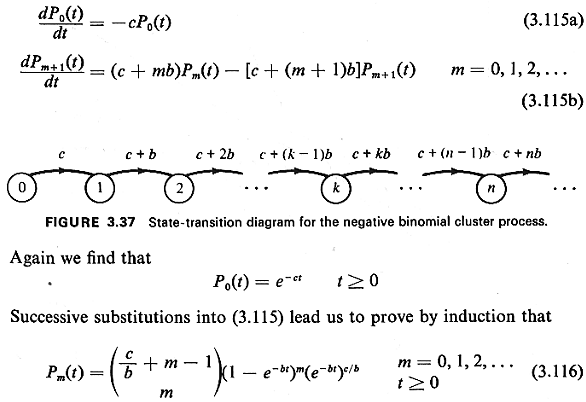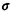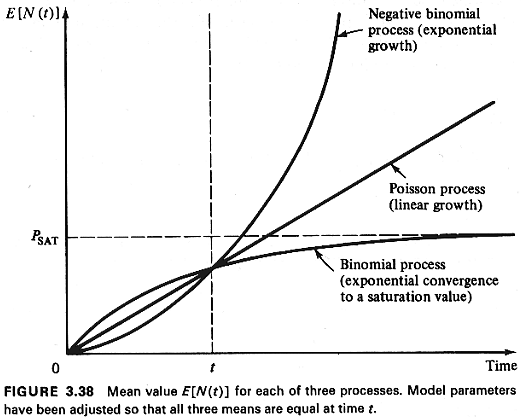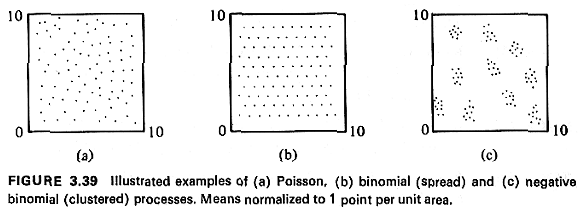## 3.9.2 Clustered Process Yielding the Negative Binomial PMF

Rogers's clustered spatial process gives rise to the negative binomial distribution. In this model we assume that a cell becomes more attractive with each additional entity that locates there. In particular, if there are m entities there at time t, new entities arrive in a (time) Poisson manner at rate c + bm (c > 0, b > 0). The state-transition diagram for this infinite-state pure birth process is shown in Figure 3.37. Proceeding as usual, the set of coupled differential equations governing this process are

``

This is the negative binomial pmf [See, for example, A. W. Drake, Fundamentals of Applied Probability, McGraw-Hill, Inc., New York, 1967, pp. 129-130,153.] with mean

```
E[N(t)] = (c/b)(ebt - 1    (3.117)

```

and variance

```2N(t) = (c/b)(ebt - 1)ebt  (3.118)

```

The ratio of the mean to the variance is

```
r = ebt		 (3.119)

```

which is always greater than unity (which is what we want with a clustered process).
Although the "increasing attractiveness" interpretation of this process is appealing for a clustering process, there are other plausible system dynamics yielding the same negative binomial pmf.
For comparative purposes, we have sketched the mean value E[N(t)] for each of the three cell occupancy laws- Poisson, binomial, negative binomial--in Figure 3.38. Note that the binomial (spread) process reaches a"saturation" population, Psat, whereas the Poisson process grows linearly in time and the negative binomial (clustered) process "explodes" at an exponential rate. Figure 3.39 illustrates each process over a 10- by 10-kilometer city.